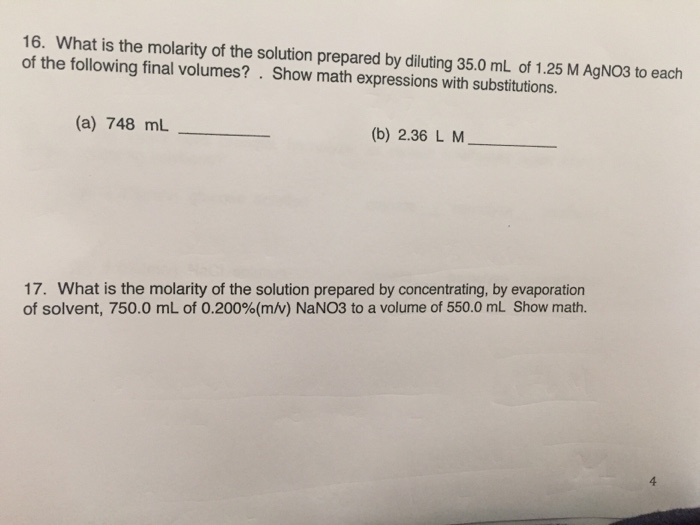# Question & Answer: 16. What is the molarity of the solution prepared by diluting 35.0 mL of 1.25 M AgNO3 to each…..

I really need help with 16 and 17! Detailed explanations would be very helpful thank you!!16. What is the molarity of the solution prepared by diluting 35.0 mL of 1.25 M AgNO3 to each of the following final volumes?. Show math expressions with substitutions. (a) 748 mL (b) 2.36 L M 17. What is the molarity of the solution prepared by concentrating, by evaporation of solvent, 750.0 mL of 0.200%(m/v) NaNO3 to a volume of 550.0 mL Show math. 4

Q16.

M = mol / Volume, by definition

Don't use plagiarized sources. Get Your Custom Essay on
Question & Answer: 16. What is the molarity of the solution prepared by diluting 35.0 mL of 1.25 M AgNO3 to each…..
GET AN ESSAY WRITTEN FOR YOU FROM AS LOW AS \$13/PAGE

Apply Dilution law:

M1*V1 = M2*V2

so…

M2 = M1*V1/V2 always true

M2 = (35 mL *1.25 M)/(748 mL)

M2 = 0.05848 M

b)

for V = 2.36 L, change to mL = 2360 mL

substitute

M2 = M1*V1/V2 always true

M2 = (35 mL*1.25 M)/(2360 mL)

M2 = 0.018538 M

Question 17.

Once again, we can use dilution law

now we use mass by volume concentrations

C1*V1 = C2*V2

where C is the ocncentration by mass and volume of the sample

this is concentrating, so expect C2 > C1

Solve for C2

C2 = C1*V1/V2

C2 = 0.2% * 750 mL/ 550 mL

C2 = 0.2727 %